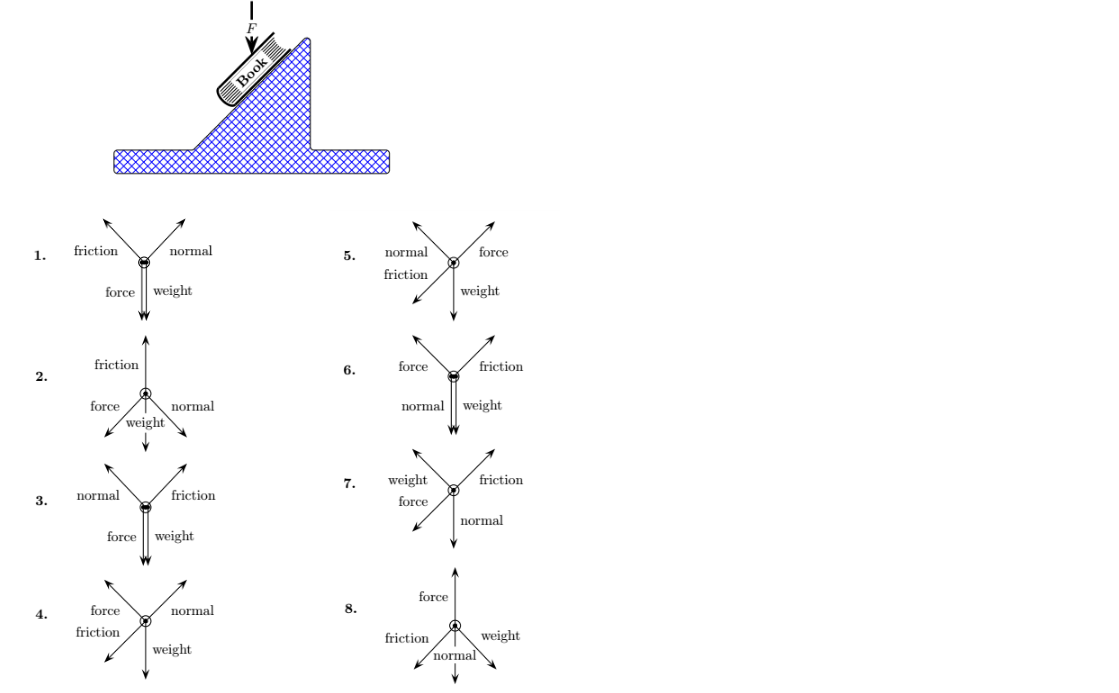Problem: A book is at rest on an incline as shown above. A constant force vertically downward is in contact with the book. The following figures show several attempts at drawing free-body diagrams for the book. Which figure has the correct directions for each force? The magnitudes of the forces are not necessarily drawn to scale.

FREE Expert Solution
83% (343 ratings)
Problem Details

A book is at rest on an incline as shown above. A constant force vertically downward is in contact with the book. The following figures show several attempts at drawing free-body diagrams for the book. Which figure has the correct directions for each force? The magnitudes of the forces are not necessarily drawn to scale.